### LSTM Forward and Backward Pass

#### Introduction

Hi, I'm Arun, a graduate student at UIUC.

While trying to learn more about recurrent neural networks, I had a hard time finding a source which explained the math behind an LSTM, especially the backpropagation, which is a bit tricky for someone new to the area. Frameworks such as Torch and Theano make life easy through automatic differentiation, which takes away the pain of having to manually compute gradient equations. However, to gain a better understanding of how things actually work and satisfy curiosity, we must dive into the details. This is an attempt at presenting the LSTM forward and backward equations in a manner which can be easily digested.

I would recommend going through A Quick Introduction to Backpropagation before proceeding further, to familiarize oneself with how backpropagation and the chain rule work, as well as the notation used in the slides that follow. Basic knowledge of neural networks, elementary calculus and matrix algebra is recommended.

### Forward Pass: Initial State### Forward Pass: Input and Gate Computation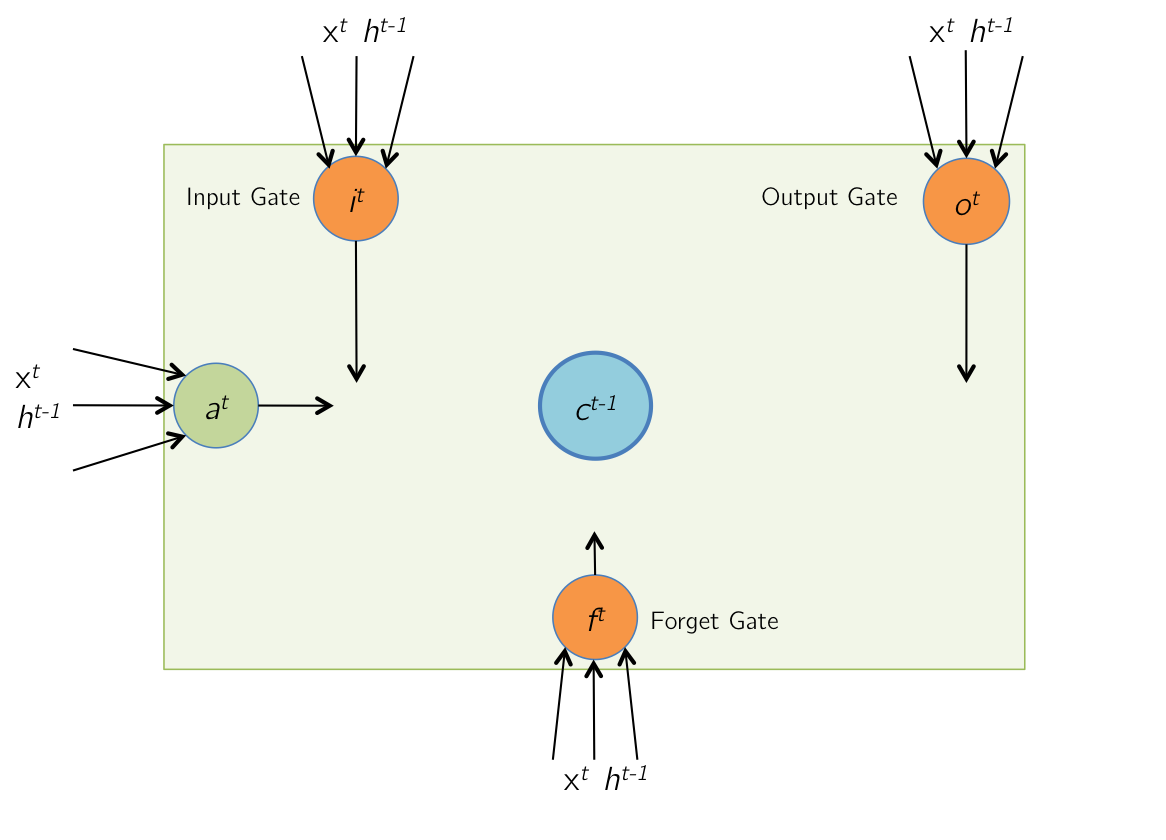\begin{align} a^t &= \tanh(W_cx^t + U_ch^{t-1}) = \tanh(\hat{a}^t) \\ i^t &= \sigma(W_ix^t + U_ih^{t-1}) = \sigma(\hat{i}^t) \\ f^t &= \sigma(W_fx^t + U_fh^{t-1}) = \sigma(\hat{f}^t) \\ o^t &= \sigma(W_ox^t + U_oh^{t-1}) = \sigma(\hat{o}^t) \end{align} Ignoring the non-linearities, \begin{align} z^t = \begin{bmatrix} \hat{a}^t \\ \hat{i}^t \\ \hat{f}^t \\ \hat{o}^t \end{bmatrix} &= \begin{bmatrix} W^c & U^c \\ W^i & U^i \\ W^f & U^f \\ W^o & U^o \end{bmatrix} \times \begin{bmatrix} x^t \\ h^{t-1} \end{bmatrix} \\ &= W \times I^t \end{align}

### Forward Pass: Memory Cell Update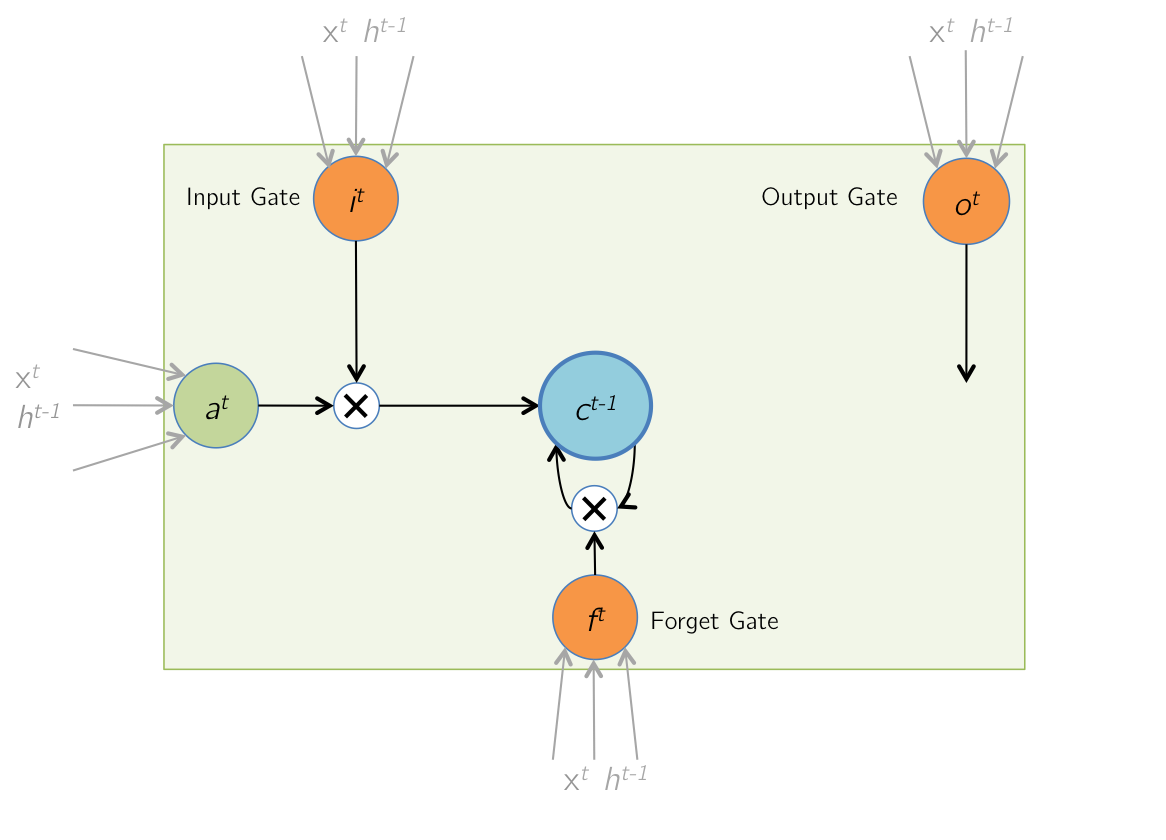$$c^t = i^t \odot a^t + f^t \odot c^{t-1}$$

### Forward Pass: Updated Memory Cells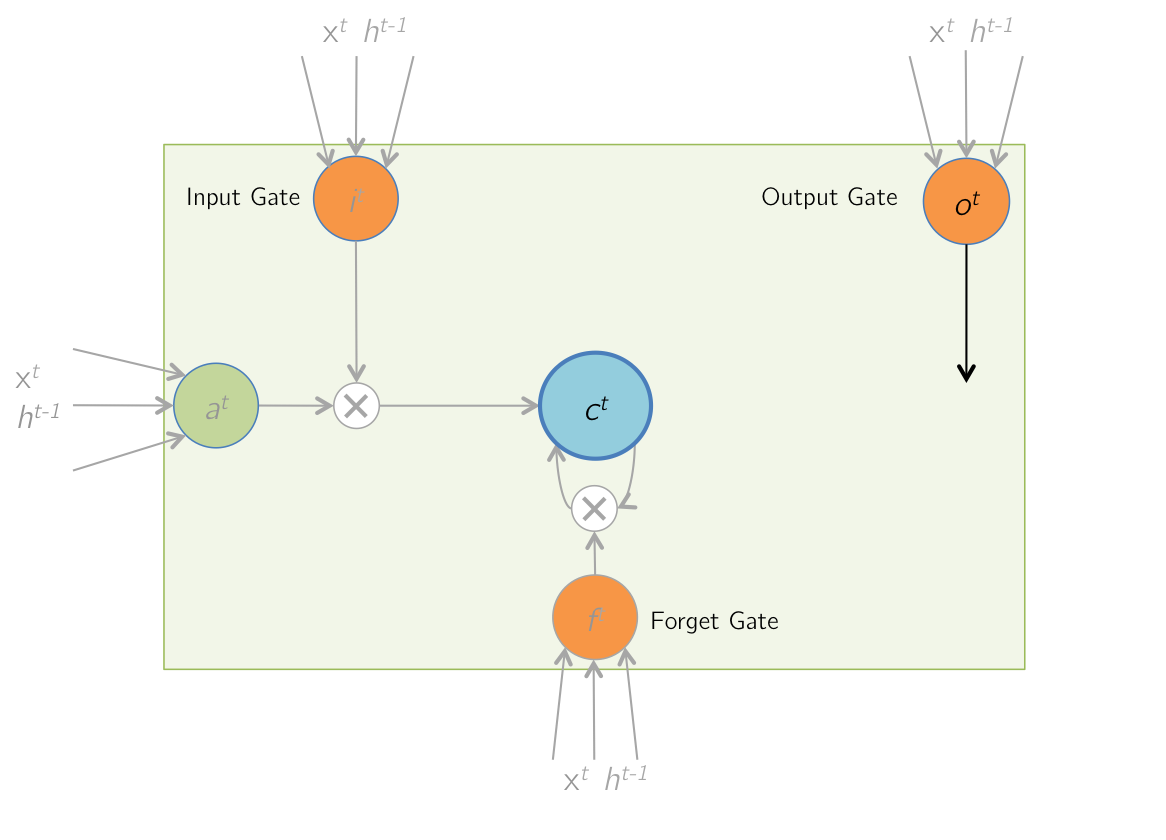$$c^{t-1} \rightarrow c^t$$

### Forward Pass: Output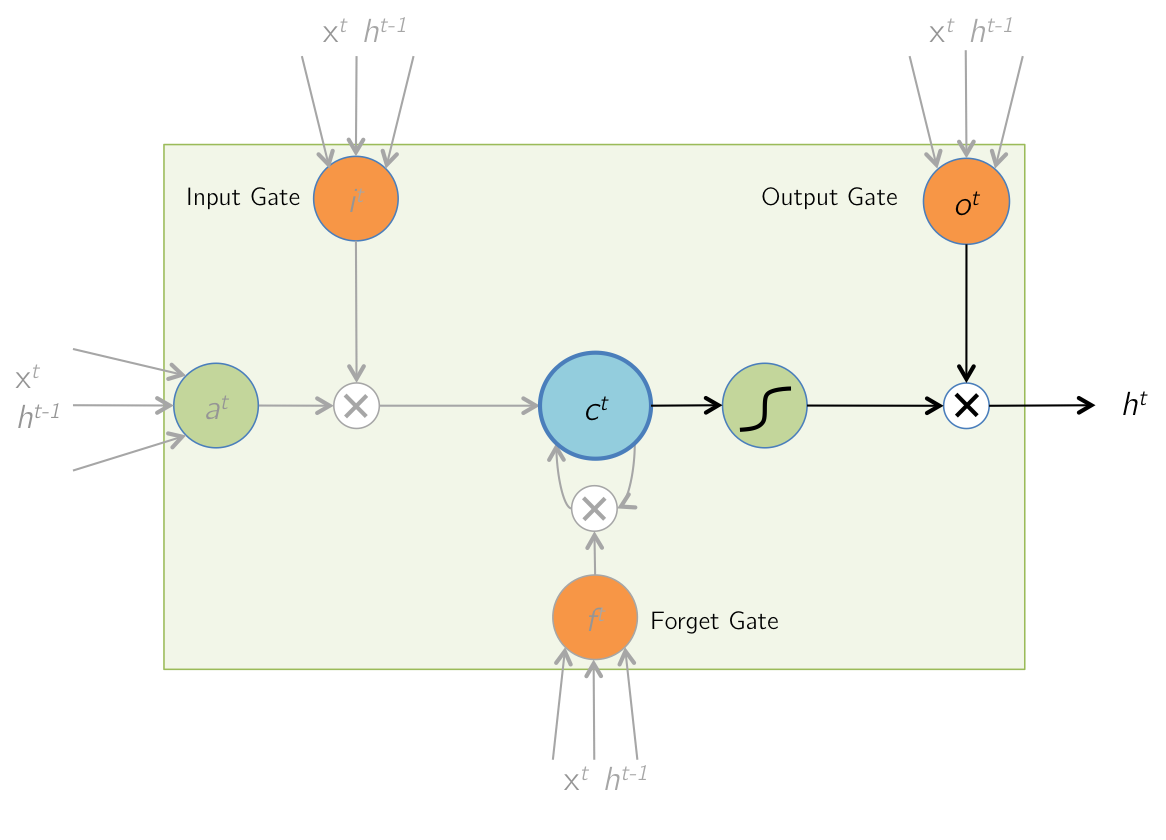$$h^t = o^t \odot \text{tanh}(c^t)$$

### Forward Pass: Unrolled Network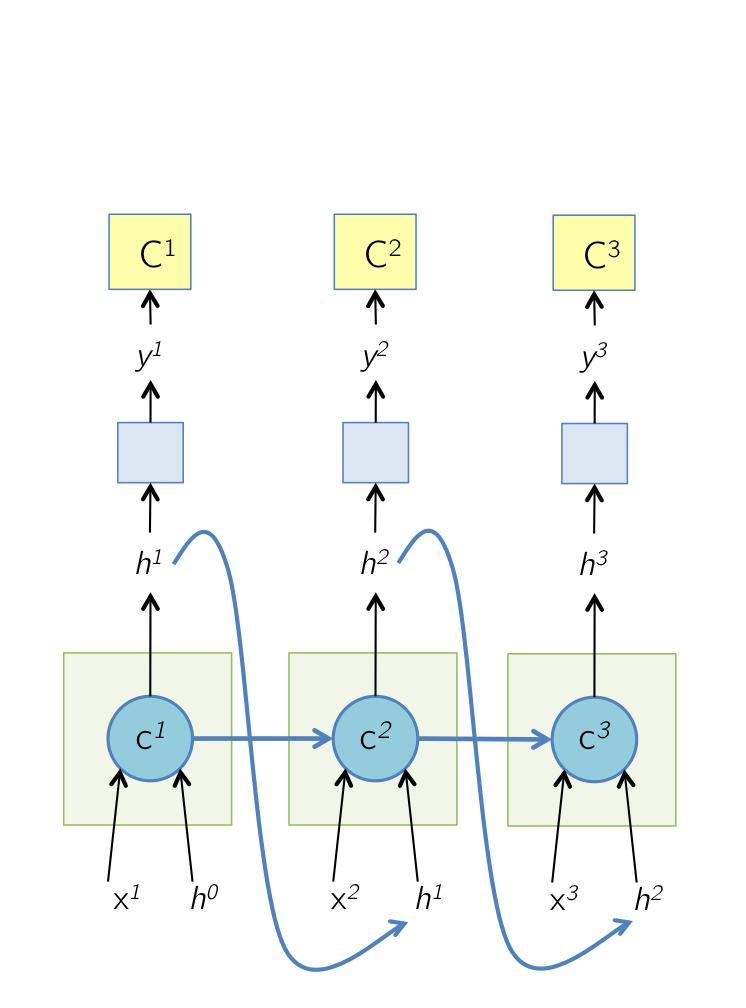### Backward Pass: Unrolled Network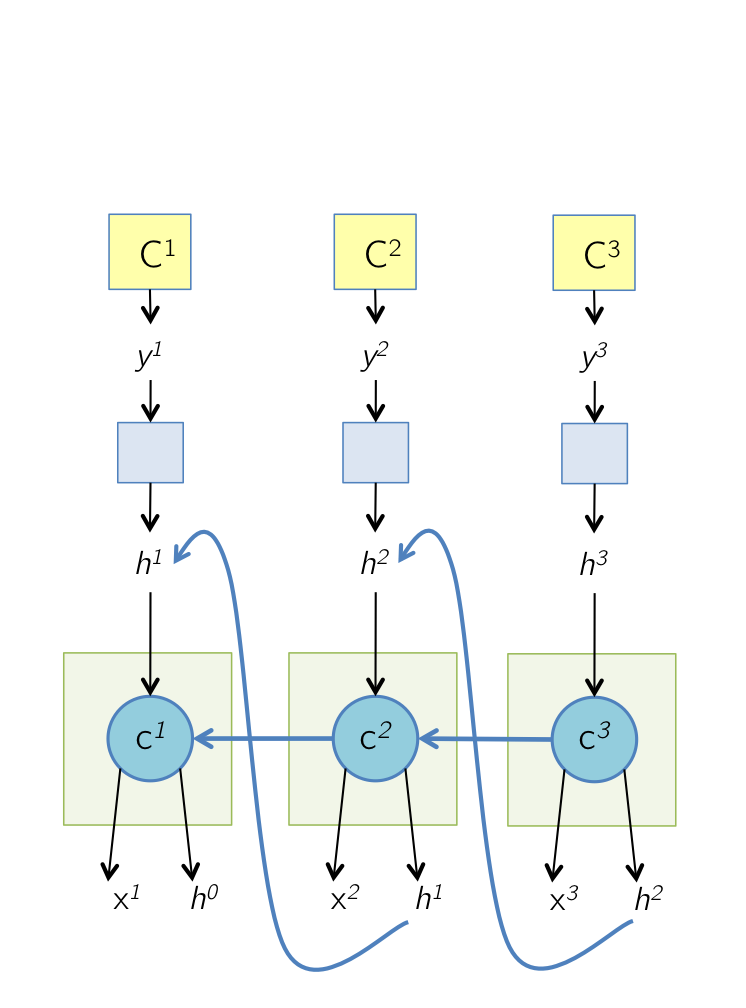### Backward Pass: Output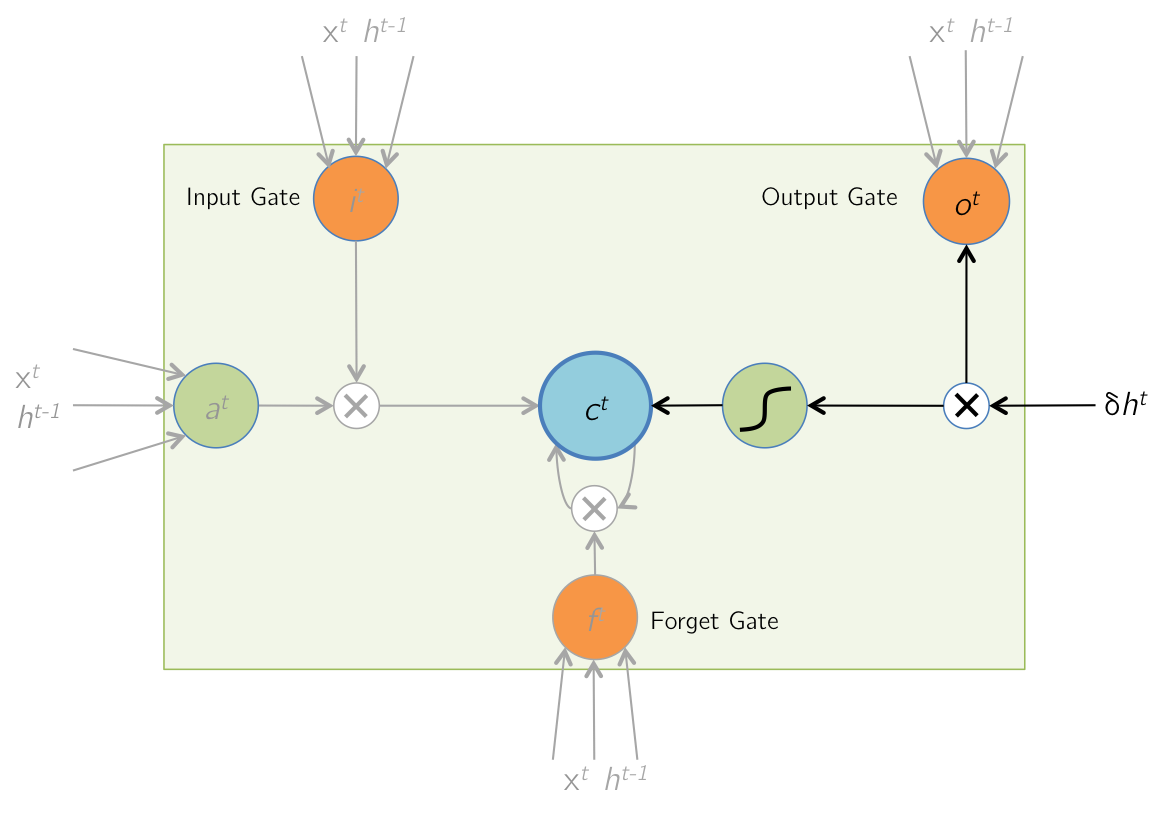\begin{align} \text{Forward Pass: } h^t &= o^t \odot \tanh(c^t) \\ \text{Given } \delta h^t &= \displaystyle\frac{\partial E}{ \partial h^t}, \text{find } \delta o^t, \delta c^t \end{align} \begin{align} \frac{\partial E}{\partial o^t_i} &= \frac{\partial E}{\partial h^t_i} \cdot \frac{\partial h^t_i}{\partial o^t_i}\\ &= \delta h^t_i \cdot \tanh(c^t_i) \\ \therefore \delta o^t &= \delta h^t \odot \tanh(c^t)\\ \\ \frac{\partial E}{\partial c^t_i} &= \frac{\partial E}{\partial h^t_i} \cdot \frac{\partial h^t_i}{\partial c^t_i}\\ &= \delta h^t_i \cdot o^t_i \cdot (1-\tanh^2(c^t_i))\\ \therefore \delta c^t & \color{red}+= \color{black}\delta h^t \odot o^t \odot (1-\tanh^2(c^t)) \end{align} Note that the $$\color{red} +=$$ above is so that this gradient is added to gradient from time step $$(t+1)$$ (calculated on next slide, refer to the gradient accumulation mentioned in the previous slide)

### Backward Pass: LSTM Memory Cell Update\begin{align} \text{Forward Pass: } c^t &= i^t \odot a^t + f^t \odot c^{t-1} \\ \text{Given } \delta c^t &= \displaystyle\frac{\partial E}{ \partial c^t}, \text{find } \delta i^t, \delta a^t, \delta f^t, \delta c^{t-1} \end{align} \begin{array}{l|l} \begin{align} \frac{\partial E}{\partial i^t_i} &= \frac{\partial E}{\partial c^t_i} \cdot \frac{\partial c^t_i}{\partial i^t_i} & \frac{\partial E}{\partial f^t_i} &= \frac{\partial E}{\partial c^t_i} \cdot \frac{\partial c^t_i}{\partial f^t_i}\\ &= \delta c^t_i \cdot a^t_i & &= \delta c^t_i \cdot c^{t-1}_i\\ \therefore \delta i^t &= \delta c^t \odot a^t & \therefore \delta f^t &= \delta c^t \odot c^{t-1}\\ \\ \frac{\partial E}{\partial a^t_i} &= \frac{\partial E}{\partial c^t_i} \cdot \frac{\partial c^t_i}{\partial a^t_i} & \frac{\partial E}{\partial c^{t-1}_i} &= \frac{\partial E}{\partial c^t_i} \cdot \frac{\partial c^t_i}{\partial c^{t-1}_i}\\ &= \delta c^t_i \cdot i^t_i & &= \delta c^t_i \cdot f^t_i \\ \therefore \delta a^t &= \delta c^t \odot i^t & \therefore \delta c^{t-1} &= \delta c^t \odot f^t \end{align} \end{array}

### Backward Pass: Input and Gate Computation - I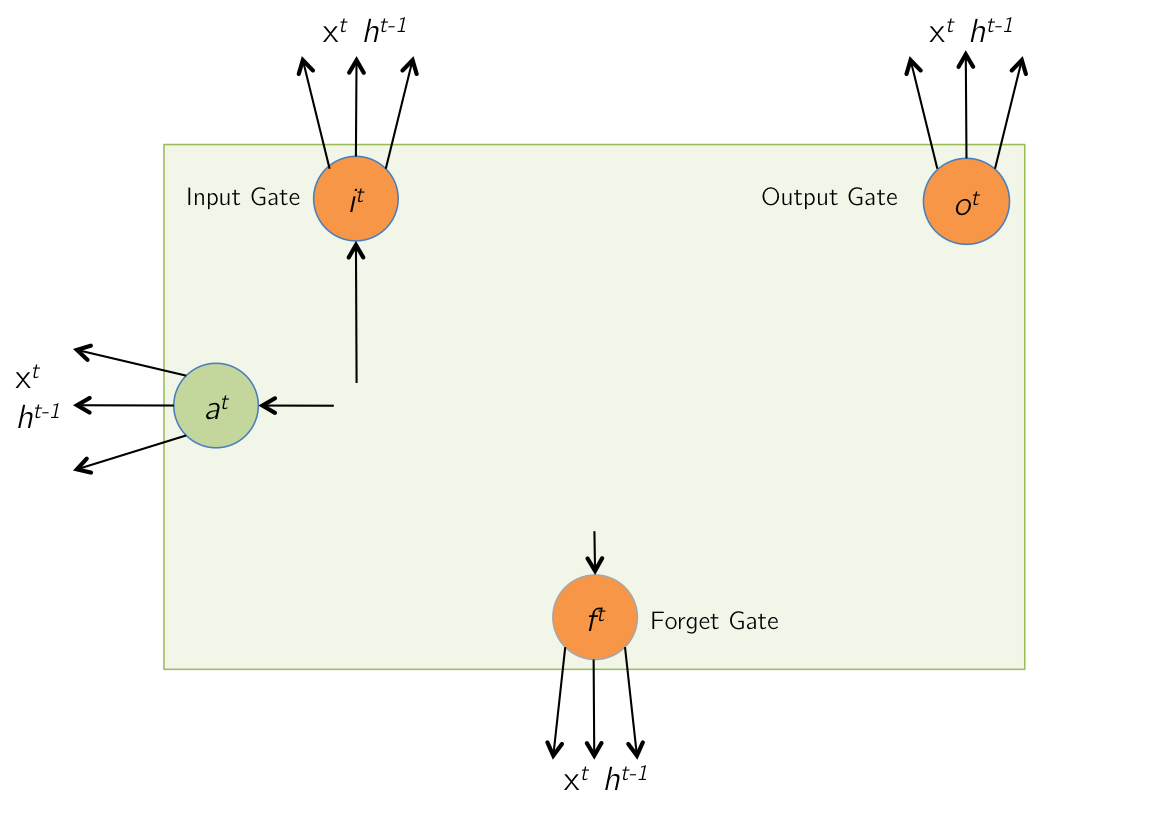\begin{align} \text{Forward Pass: } z^t &= \begin{bmatrix} \hat{a}^t \\ \hat{i}^t \\ \hat{f}^t \\ \hat{o}^t \end{bmatrix} = W \times I^t \\ \text{Given } \delta a^t, \delta i^t , &\delta f^t, \delta o^t, \text{ find } \delta z^t \end{align} \begin{array}{l|l} \begin{align} \delta \hat{a}^t &= \delta a^t \odot (1-\tanh^2(\hat{a}^t)) \\ \delta \hat{i}^t &= \delta i^t \odot i^t \odot (1-i^t) \\ \delta \hat{f}^t &= \delta f^t \odot f^t \odot (1-f^t) \\ \delta \hat{o}^t &= \delta o^t \odot o^t \odot (1-o^t) \\ \delta z^t &= \left[ \delta\hat{a}^t, \delta\hat{i}^t, \delta\hat{f}^t, \delta\hat{o}^t \right]^T \end{align} \end{array}

### Backward Pass: Input and Gate Computation - II\begin{align} \text{Forward Pass: } z^t &= W \times I^t \\ \text{Given } \delta z^t, \text{ find }& \delta W^t, \delta h^{t-1} \end{align} \begin{align} \delta I^t &= W^T \times \delta z^t\\ \text{As } I^t &= \begin{bmatrix} x^t \\ h^{t-1} \end{bmatrix} \text{, }\\ \delta h^{t-1} &\text{ can be retrieved from } \delta I^t\\ \delta W^t &= \delta z^t \times (I^t)^T\\ \end{align}

### Parameter Update

If input $$x$$ has $$T$$ time-steps, i.e. $$x = [ x^1, x^2, \cdots, x^T ]$$, then \begin{align} \delta W &= \sum_{t = 1}^T \delta W^t \\ \end{align} $$W$$ is then updated using an appropriate Stochastic Gradient Descent solver.